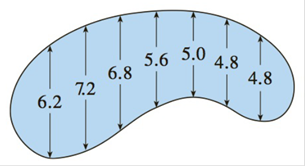Chapter 7.7, Problem 30E

Chapter
Section
Textbook Problem

# The widths (in meters) of a kidney-shaped swimming pool were measured at 2-meter intervals as indicated in the figure. Use Simpson’s Rule to estimate the area of the pool.To determine

To evaluate: To use Simpson’s rule it estimate the area of a part where width is measured at 2 meter interval.

Explanation

Formula used:

Simpson’s rule:

abfxdx=b-a3nfx0+4fx1+2fx2+4fx3.

### Still sussing out bartleby?

Check out a sample textbook solution.

See a sample solution

#### The Solution to Your Study Problems

Bartleby provides explanations to thousands of textbook problems written by our experts, many with advanced degrees!

Get Started

#### In Exercise 11-14, factor the expression. x2+5x+6

Calculus: An Applied Approach (MindTap Course List)

#### What is the inverse of a matrix A?

Finite Mathematics for the Managerial, Life, and Social Sciences

#### Determine 3.

Mathematics For Machine Technology